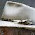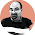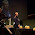### Some quotations about the amplituhedron

posted by @ulaulaman via @tanzmax about #amplituhedronHowever, the calculation using the shape in an infinite-dimensional space, the amplituhedron, should provide us with completely new perspectives how to look at the dynamics – perspective that is timeless, obscures the location of objects and events in the space and time, and obscures the unitarity (the requirement that the total quantum-calculated probability of all possibilities remains 100%), but it unmasks some other key structures that dictate what the probabilities should be, structures we were largely ignorant about.
If the new picture becomes sufficiently generalized, you could perhaps throw away the old books because you will get an entirely new framework to compute these things and to think about all these things. But once again, you don't have to throw them away because the physical results are the same.
(Luboš Motl on physics.stackexchange.com)
The amplituhedron is not built out of space-time and probabilities; these properties merely arise as consequences of the jewel’s geometry. The usual picture of space and time, and particles moving around in them, is a construct.
(from Quanta Magazine via Marginal Revolution)
This rich structure is also completely new to the mathematicians.
(Jaroslav Trnka - pdf)
We establish a direct connection between scattering amplitudes in planar four-dimensional theories and a remarkable mathematical structure known as the positive Grassmannian. The central physical idea is to focus on on-shell diagrams as objects of fundamental importance to scattering amplitudes. We show that the all-loop integrand in N=4 SYM is naturally represented in this way. On-shell diagrams in this theory are intimately tied to a variety of mathematical objects, ranging from a new graphical representation of permutations to a beautiful stratification of the Grassmannian G(k,n) which generalizes the notion of a simplex in projective space. All physically important operations involving on-shell diagrams map to canonical operations on permutations; in particular, BCFW deformations correspond to adjacent transpositions. Each cell of the positive Grassmannian is naturally endowed with positive coordinates and an invariant measure which determines the on-shell function associated with the diagram. This understanding allows us to classify and compute all on-shell diagrams, and give a geometric understanding for all the non-trivial relations among them. Yangian invariance of scattering amplitudes is transparently represented by diffeomorphisms of G(k,n) which preserve the positive structure. Scattering amplitudes in (1+1)-dimensional integrable systems and the ABJM theory in (2+1) dimensions can both be understood as special cases of these ideas. On-shell diagrams in theories with less (or no) supersymmetry are associated with exactly the same structures in the Grassmannian, but with a measure deformed by a factor encoding ultraviolet singularities. The Grassmannian representation of on-shell processes also gives a new understanding of the all-loop integrand for scattering amplitudes, presenting all integrands in a novel dLog form which directly reflects the underlying positive structure.
(the abstract of the work)
See the Nima's talk at SUSY 2013 in Italy

#### 6 comments:

1.Wow, after seeing the image above, at reduced size, TPP was just sure the diagram was about hydrophyte stem anatomy. See for yourself here.

1.Wonderful!!! It seems that the mathematics of amplituhedron could be fundamental not only for physics!

2.How do we distinguish between this being deep and fundamental, or merely a computational shortcut?

1.If using this computational shortcut we are able to find a model that is more fundamental than standard model, for example

3.What is the source of this image of the Amplituhedron? Thank you!
https://lh3.googleusercontent.com/-rm5V_ppFWm0/UjsKC0e4vfI/AAAAAAAAIm0/mb1oNFdUQXY/s512/20130919-amplituhedron.jpg

1.The last paper that I linked: "Scattering Amplitudes and the Positive Grassmannian"

Markup Key:
- <b>bold</b> = bold
- <i>italic</i> = italic
- <a href="http://www.fieldofscience.com/">FoS</a> = FoS